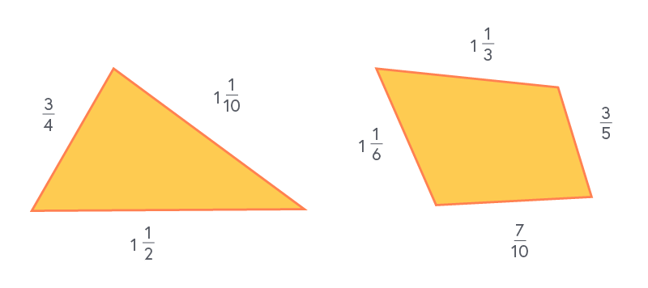4.10

# Working with three or more fractions

In this step we work through two further problems where three or more fractions are added together.

## Example

Which of the two shapes below has the largest perimeter? The units for each side are the same and the drawing is not to scale.### Triangle perimeter

First, convert $1\frac{1}{10}$ to $\frac{11}{10}$, and convert $1\frac{1}{2}$ to $\frac{3}{2}$, so we are not working with mixed numbers.

The perimeter is equal to $\frac{3}{4} + \frac{11}{10} + \frac{3}{2}$.

To add these fractions, we look for the lowest common denominator across all three fractions, which is 20.

$Perimeter_{T} = \frac{15}{20} + \frac{22}{20} + \frac{30}{20}$ $= \frac{67}{20}$ $= 3\frac{7}{20}$

First, again to change from mixed numbers, convert $1\frac{1}{6}$ to $\frac{7}{6}$, and convert $1\frac{1}{3}$ to $\frac{4}{3}$.

The perimeter is the sum of all sides, which is $\frac{7}{6} + \frac{4}{3} + \frac{3}{5} + \frac{7}{10}$.

We look for the lowest common denominator for all four fractions, which is 30.

$Perimeter_{Q} = \frac{35}{30} + \frac{40}{30} + \frac{18}{30} + \frac{21}{30}$ $= \frac{114}{30}$ $= 3\frac{24}{30}$ $= 3\frac{4}{5}$

So both are ‘3 and something’. We need to compare the fractional parts of the number. To do this, we again find the lowest common denominator:

$\frac{7}{20} = \frac{7}{20}$ and $\frac{4}{5} = \frac{16}{20}$

$3\frac{4}{5}$ is bigger than $3\frac{7}{20}$, so the quadrilateral has the larger perimeter.

Next week we’ll be asking you to create and complete calculations with three or more fractions and different operators.